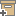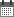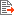ניהול
קהילה:
אסיף מאגר המחקר החקלאי
Determination of robotic melon harvesting efficiency: a probabilistic approachBack to searchPrevious item
Next item
Year:
2015
Authors :
ציון, בועז
;
.
Volume :
54
Co-Authors:

Moshe Mann,
Boaz Zion,
Itzhak Shmulevich
Dror Rubinstein

Facilitators :
From page:
0
To page:
0
(
Total pages:
1
)
Abstract:

To automate the harvesting of melons, a mobile Cartesian robot is developed that traverses at a constant velocity over a row of precut melons whose global coordinates are known. The motion planner is programmed to have the robot harvest as many melons as possible. Numerous simulations of the robot over a field with different sets of randomly distributed melons resulted in nearly identical percentages of melons harvested. This result holds true over a wide range of robot dimensions, motor capabilities, velocities and melon distributions. Using probabilistic methods, we derive these results by modelling the robotic harvesting procedure as a stochastic process. In this simplified model, a harvest ratio is predicted analytically using Poisson and geometric distributions. Further analysis demonstrates that this model of robotic harvesting is an example of an infinite length Markov chain. Applying the mathematical tools of Markov processes to our model yields a formula for the harvest percentage that is in strong agreement with the results of the simulation. The significance of the approach is demonstrated in two of its applications: to select the most efficient actuators for maximal melon harvesting and determine the set of optimal velocities along a row of melons of varying densities.

Note:
Related Files :
geometric distribution
Harvesting robots
Markov chain
performance prediction
probabilistic analysis
עוד תגיות
תוכן קשור
More details
DOI :
10.1080/00207543.2015.1081428
Article number:
0
Affiliations:
Database:
Publication Type:
מאמר
;
.
Language:
אנגלית
Editors' remarks:
ID:
49939
Last updated date:
02/03/2022 17:27
Creation date:
13/09/2020 23:10Scientific Publication
Determination of robotic melon harvesting efficiency: a probabilistic approach
54

Moshe Mann,
Boaz Zion,
Itzhak Shmulevich
Dror Rubinstein

Determination of robotic melon harvesting efficiency: a probabilistic approach

To automate the harvesting of melons, a mobile Cartesian robot is developed that traverses at a constant velocity over a row of precut melons whose global coordinates are known. The motion planner is programmed to have the robot harvest as many melons as possible. Numerous simulations of the robot over a field with different sets of randomly distributed melons resulted in nearly identical percentages of melons harvested. This result holds true over a wide range of robot dimensions, motor capabilities, velocities and melon distributions. Using probabilistic methods, we derive these results by modelling the robotic harvesting procedure as a stochastic process. In this simplified model, a harvest ratio is predicted analytically using Poisson and geometric distributions. Further analysis demonstrates that this model of robotic harvesting is an example of an infinite length Markov chain. Applying the mathematical tools of Markov processes to our model yields a formula for the harvest percentage that is in strong agreement with the results of the simulation. The significance of the approach is demonstrated in two of its applications: to select the most efficient actuators for maximal melon harvesting and determine the set of optimal velocities along a row of melons of varying densities.

Scientific Publication
You may also be interested in